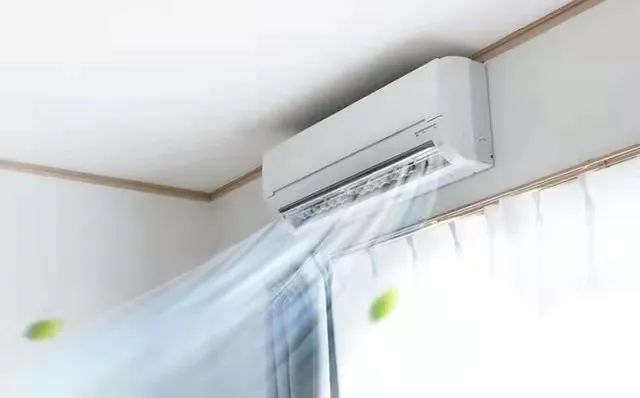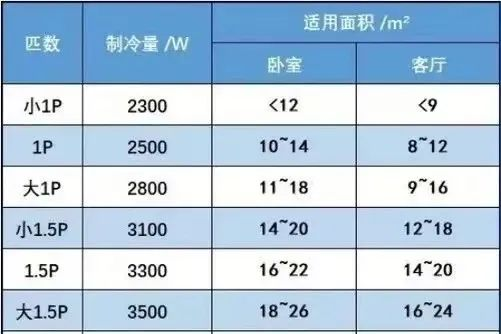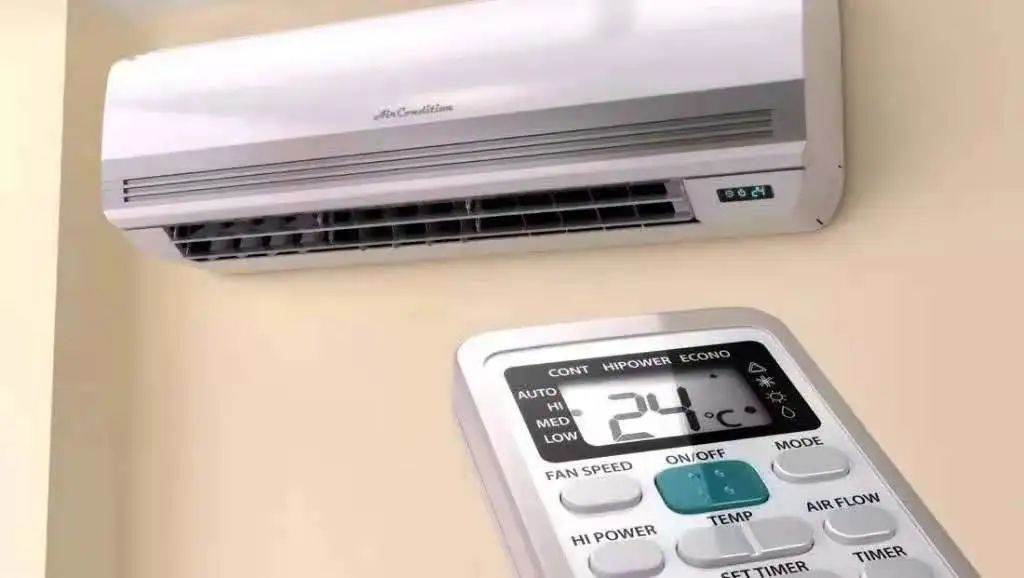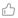# 计量科普 | 炎炎夏日，怎样才能买到合适的空调？

2022-06-29 10:07:5115m²:  130*15=1950（W）

20m²：130*20=2600（W）

25m²：130*25=3250（W）

28m²：130*28=3640（W）

30m²：160*30=4800（W）

40m²：160*40=6400（W）

45m²：160*45=7200（W）1.关于单位

1 kcal/h = 1.163 W

1 kw = 860 kcal/h

1 HP = 735 W

1 RT = 3.516 kW= 3024 kcal/h

1MPa = 1000 KPa = 10 kg/cm²

1bar = 1kg/cm² = 0.1 MPa = 14.70 psi

2.关于加氟

3.关于能效比

(责任编辑 龚晓艺）0